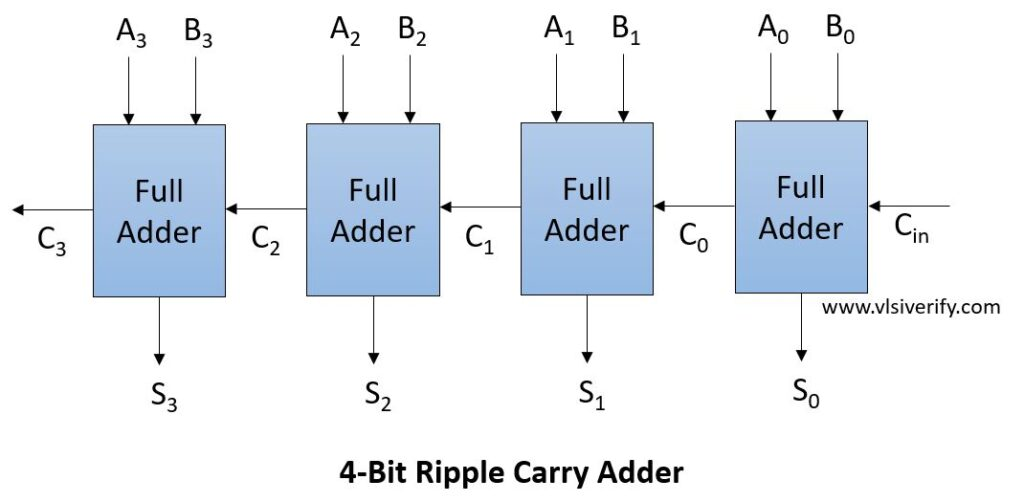Tutorials

As we discussed full adder can add single bit two inputs and extra carry bit generated from its previous stage. To add multiple ‘n’ bits binary sequence, multiples cascaded full adders can be used which can generate a carry bit and be applied to the next stage full adder as an input till the last stage of full adder. This appears as carry-bit ripples to the next stage, hence it is known as “Ripple carry adder”.

Worst case delay = [(n-1) full adder * carry propagation delay of each adder] + sum propagation delay of each full adder

## Block DiagramA carry bit ripples to the next stage, all stages of full adders are not simultaneously used. Each full adder stage needs to be generated first before it can add the next stage two numbers, thus it introduces propagation delay in computation. This is why the ripple carry adder is slower.

Due to its simple layout and less design complexity, it allows for fast design time.

## Ripple Carry Adder Verilog Code

``````module full_adder(
input a, b, cin,
output sum, cout
);

assign {sum, cout} = {a^b^cin, ((a & b) | (b & cin) | (a & cin))};
//or
//assign sum = a^b^cin;
//assign cout = (a & b) | (b & cin) | (a & cin);
endmodule

module ripple_carry_adder #(parameter SIZE = 4) (
input [SIZE-1:0] A, B,
input Cin,
output [SIZE-1:0] S, Cout);

genvar g;

full_adder fa0(A, B, Cin, S, Cout);
generate  // This will instantiate full_adder SIZE-1 times
for(g = 1; g<SIZE; g++) begin
full_adder fa(A[g], B[g], Cout[g-1], S[g], Cout[g]);
end
endgenerate
endmodule
``````

### Testbench Code

``````module RCA_TB;
wire [3:0] S, Cout;
reg [3:0] A, B;
reg Cin;

ripple_carry_adder rca(A, B, Cin, S, Cout);

initial begin
\$monitor("A = %b: B = %b, Cin = %b --> S = %b, Cout = %b, Addition = %0d", A, B, Cin, S, Cout, add);
A = 1; B = 0; Cin = 0; #3;
A = 2; B = 4; Cin = 1; #3;
A = 4'hb; B = 4'h6; Cin = 0; #3;
A = 5; B = 3; Cin = 1; #3;
\$finish;
end

initial begin
\$dumpfile("waves.vcd");
\$dumpvars;
end
endmodule``````

Output:

``````A = 0001: B = 0000, Cin = 0 --> S = 0001, Cout = 0, Addition = 1
A = 0010: B = 0100, Cin = 1 --> S = 0111, Cout = 0, Addition = 7
A = 1011: B = 0110, Cin = 0 --> S = 0001, Cout = 1, Addition = 17
A = 0101: B = 0011, Cin = 1 --> S = 1001, Cout = 0, Addition = 9``````

Verilog Codes